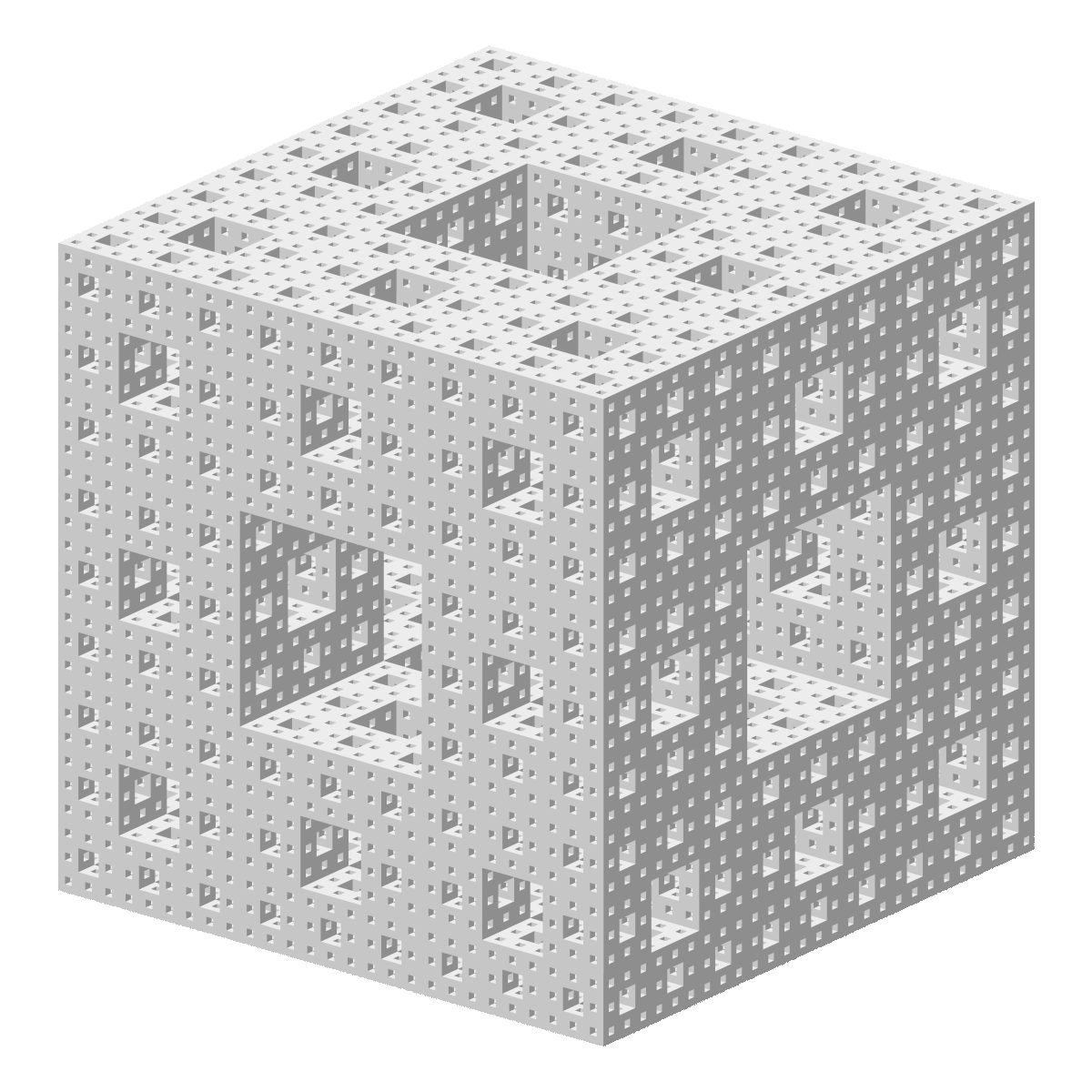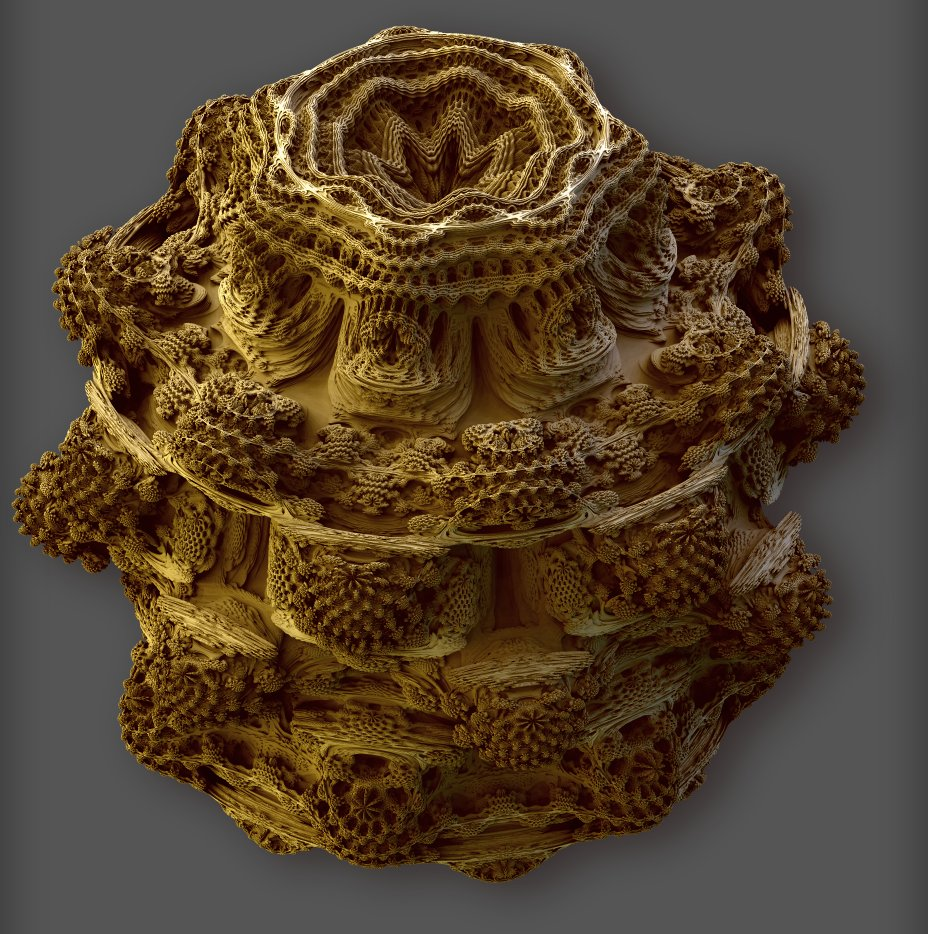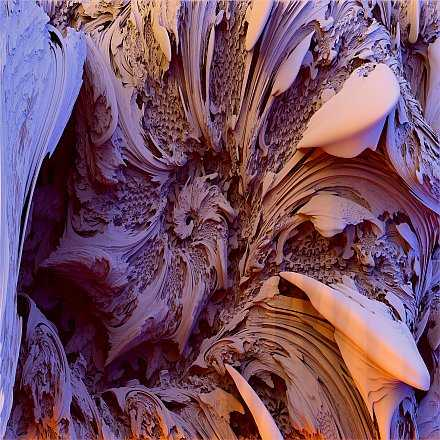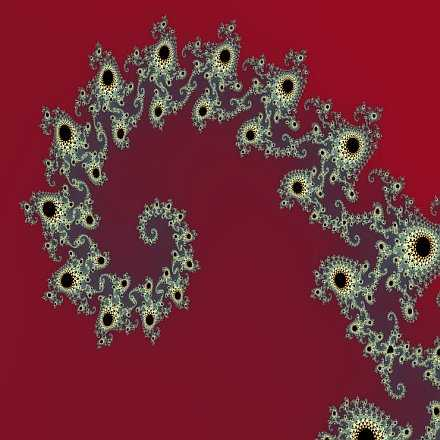# Fractals

Fractals are a relatively new mathematical concept which are shapes that have detail at all levels. (i.e., you can keep zooming into the shape and always find new patterns) Fractals originated a few million years ago, but they have only been named and studied for less than 200 years, and less than 50 years if you don’t count the times when mathematicians were calling them “Monsters”.

The most simple type of fractal is one where you take a shape, and then turn the shape into a number of smaller copies of itself. Probably the most famous example of this technique is the Sierpinski Gasket, created by taking one triangle, and turning it into 3 smaller copies of itself:As you can see, at each succeeding iteration the new triangles are replaced, and so on. The same fractal can be made by progressively taking triangles out of triangles, and both ways can be easily done using some triangular graph paper.

A nearly as well-known fractal as the Sierpinski Gasket is the Sierpinski Carpet. To make this fractal, you take a square, divide it into 9, remove its center square, and repeat. Naturally, the same can be done by adding: Divide a square into 9 and replace each part by itself:Iterations of the Sierpinski carpet

It’s possible to, as well as creating 2D self-similar fractals, to create 1-D self-similar fractals, which will be a line bending into two dimensions. An example of this would be the Koch Curve, discovered in 1904 by Niels Fabian Helge von Koch. To make it, start with a line, divide it into 3, and replace the middle section by 2 line segments. This can be shown better by the “generator” for the Koch curve, which shows the before and after:If you repeat these steps, you’ll generate the following picture:This fractal was originally called a “monster” due to the fact that it is continuous everywhere (there are no holes), but it is impossible to take a slope at a single point. In this way it is a shape that has no corners, which mathematicians regarded at the time as ridiculous. A similar fractal discovered earlier by the Italian mathematician Guiseppe Peano in 1890, the Peano curve, is similar but manages to fill space:This was regarded as crazier by the mathematicians than the future Koch curve- Is it a line, or is it a square? It’s actually a schematic for converting one dimension into two! Even crazier than that was the Hilbert curve, discovered by David Hilbert in 1891:It consists mainly of taking the previous curve, adding 3 copies, and connecting those copies together.

Some of these 1-dimensional curves can be made 2-dimensional by taking multiple copies of them and putting them together. For example, there’s the Koch Snowflake, which comes from 3 copies of the Koch Curve:Surprisingly enough, the total area of the Koch Snowflake  is not some infinite series, but rather 8/5 the area of the original triangle! Some 1-dimensional curves, such as the Peano curve, don’t need to be joined to seem to be 2-dimensional. However, the Peano curve creates what seems to be a square, and a square is certainly not a fractal. The Flowsnake, discovered by Bill Gosper and later renamed to “Peano-Gosper curve” achieves the object of having a bounding area that is both fractal and tileable!# 3-Dimensional “Simple” Fractals

Up to this point we have been taking about 1 and 2-dimensional fractals, but in an effort not to make fractals too easy for computers, we now turn to 3-dimensional self-similar fractals.  To start off, the Sierpinski Carpet can be turned into a 3-dimensional version simply by replacing the squares with cubes. This causes us to use 20 times as many cubes for each step, but if you happen to have that much wood and glue around the house, you can easily make it by replacing each cube with 20 cubes, in the fashion shown below. This creates an object called the Menger Sponge, but you aren’t likely to see it on the sponge market anytime soon:The sponge pictured above is a level 4 sponge, which would take 160,000 cubes to create. 8,000 is a much more manageable number, and so Dr. Jeannine Mosely decided to create a Level 3 Menger Sponge- out of business cards. There would be 6 cards per cube, which would then be linked, and finally paneled for a grand total of 66,048 business cards, which Dr. Mosely managed to create quite a while later:As you can see, it’s large enough to crawl into, but as fun as it may seem, Dr. Mosely says that a Level 4 sponge made out of business cards would simply not be possible to make without structural support.

The Sierpinski Gasket also has a 3-dimensional analog: The Sierpinski Tetrahedron. To make it, you take the previous level, make 3 more copies of it, and join them by the corners.George Hart has made a 3D model of it, and even has a good description of how to make it in his Math Monday post. With all this, you might think that there would be an interesting 3D version of the Koch Snowflake. However, when doing it like you normally would (tetrahedra on triangle) you get something quite unexpected…Now join 4 of those together, and you get a cube. From a fractal.

All this time we have been referring to fractals as “1-dimensional”, “2D”, and “in 3 Dimensions” when in fact, as we have seen, they clearly aren’t. The Peano 1D fractal may as well be a square, and the Sierpinski 2D fractal may as well be a series of lines, and the Hilbert curve is somewhere in between. To make deciding easier, Felix Hausdorff and Abram Samoilovitch Besicovitch invented the concept of fractal dimension, which can not only be an integer, but also any number in between. To compute the fractal dimension, we use the formula D=Log(N)/Log(l), where N is the number of pieces that replace the original shape, and l is one over the scale factor. (The base of the logarithm does not matter) For example, in the Sierpinski gasket, N=3, and l=2, which means that D=Log(3)/Log(2), or about 1.584962501. This means that the Sierpinski gasket is slightly less than a square, and quite a bit more than a line. Similarly, for the “3D” Menger Sponge, its fractal dimension is Log(20)/Log(3)=2.726833028. Finally, the fractal dimension of the Peano curve is Log(9)/Log(3)= exactly 2, which means that the Peano curve, at the ∞th iteration, may as well be a square. A large list of fractals and their fractal dimensions can be found at Wikipedia.

## Fractals Imitating Reality, or Vice Versa?

Self-similar fractals are not just an abstraction, though. Many plants, such as cauliflower and broccoli show self-similar behavior. On cauliflower, it can be seen by the lobes of the surface, and broccoli is a bit more chaotic, but still shows the same behavior:Trees can also be simulated reasonably well using fractals:

# Escape-Time Fractals

Self-similar fractals get a bit boring after a while, so let’s explore another kind of fractal: Escape-time fractals. Escape-time fractals take place on the complex plane, which is an extension of the real line. Basically, it’s a plane of numbers of the form x+i*y, where i is the “imaginary number” sqrt(-1). Imaginary numbers (a+i*y) can have all the operations of real numbers done to them, such as addition (a+i*b+c+i*d=(a+c)+i(b+d)), multiplication ((a+i*b)*(c+i*d)=(ac-bd)+i(bc+ad)), as well as division, square roots, exponentiation, and every other function that can be applied to the real numbers. Now, consider a function repeatedly applied to an initial complex number until the point escapes a circle of radius r, and color it according to the number of iterations it takes to escape from the circle. If the point never escapes, or doesn’t escape after however many iterations, then color the point black.

Pierre Fatou and Gaston Julia first investigated, in the 1910s, iterations of this type, specifically iterations of the type z->z^2+c, where c is the initial point, and z is the point that changes. However, they simply noted the chaos of the system: Julia studied variations where c was a single number and z was anything, and Fatou studied the function for where c was a single number and where the initial value of z was 0. It wasn’t until 1979 that Benoit Mandelbrot would expand on Julia’s work using computer analysis.

Mandelbrot decided to use a computer to plot Julia sets using the color-by-point method, and was stunned by the results. Julia sets create self-similar fractals, but are much more interesting as they use color and are much more varied, as the following video of a set of Julia sets shows:

The next logical step after studying these would be to let c be equal to the initial value of z, and so he created what is now known as the Mandelbrot Set.(right click and press view image for full size)

The Mandelbrot Set is unlike any of the fractals that we’ve come across so far in that it has no self-symmetry. Although there may be shapes farther in that look like the Mandelbrot Set, they aren’t quite the whole. There are many areas of the Mandelbrot set, such as the antennae-like left regions explored in the video “Trip to E214” e.g. 10^214 zoom, so large that the smallest particles postulated by physics would be nearly a googol times larger than the universe:

Or into the “Seahorse Valley” (I am not making these names up) ,where there are intricate spiral structures:

Or into a whole host of other places by using a fractal software such as the ones at the end of this post.

An interesting thing about the Mandelbrot Set is that, the farther you zoom into an area, the more it seems to look like the corresponding Julia set for that point, such as in this video, also into the Seahorse Valley area of the Mandelbrot Set:

What’s also interesting about the Mandelbrot Set is that no matter how far you zoom in, there always appears to be more intricate structures, which has led to the rise of groups specializing in computer zooms really far into the set. An example of this is the Trip to E214, and I believe the record for high definition is 10^275:

and for low definition there’s 10^999:

New structures can even pop up deep into the set, such as a long string of Xs:

(For those who don’t have the patience to watch the above video, the point can be seen at http://stardust4ever.deviantart.com/art/XX-Reactor-Core-Deep-Zoom-131573460)

By now you should have noticed a really interesting optical illusion: When you look away from a video, the space seems to be shrinking in!  It’s also the sign not to watch all of the videos all at once.

The fractal dimension for the Mandelbrot Set can also be computed, but it’s quite complicated. In fact, it was not until 1991 that Mitsuhiro Shishikura proved that the Hausdorff dimension of the Mandelbrot set equals… 2. The area of the Mandelbrot Set, however, is not so simple. Although nobody has figured out a way to calculate it precisely (The best formula I know of (i.e. only) is given in equations 7-10 on the Mathworld page), it is possible to get an estimate of it by counting pixels from -2+-2i to 2+2i, and finding what percent of them are in the set. The current best known value for the area is 1.50659177 +- 0.00000008 , given by Robert Munafo in 2003 on his page “Area of the Mandelbrot Set“. Cyril Soler, a researcher at the National de Recherche en Informatique et Automatique, conjectures that the value is exactly sqrt(6*pi-1)-e, but whether he is right or wrong is not known. It is also possible to calculate exact mathematical formulae for some of the subregions of the Mandelbrot Set, such as the large cardioid-shaped blob, which can be expressed in parametric form asThe Mandelbrot Set is also connected, which means that if you take any point inside the set, you can get to any other point inside the set by following some series of pathways.

Lastly, for programmers, you can easily make your own Mandelbrot set generator even if your programming language does not support complex numbers by iterating z_realtemp->z_real*z_real – z_imag*z_imag + c_real, z_imag->2*z_real*z_imag + c_imag, and z_real->z_realtemp.A good pseudocode example is at http://en.wikipedia.org/wiki/Mandelbrot_set#Computer_drawings.

Naturally, the Mandelbrot Set is not the only escape-time fractal there is. First of all, there are the Mandelbrot Generalizations, z->z^p+c: (0<p<20 for the video)

There’s also the Phoenix Julia set, which not only relies on the previous point but the point before that, zn + 1 = zn2 + Re(c) + Im(c) * zn – 1, , where c is constant:

A good online program for exploring it on your own is at http://www.jamesh.id.au/fractals/mandel/Phoenix.html

There are an infinitude of others, so I won’t go through them all here, but a good gallery of escape-time fractal art is at http://fractalarts.com/ASF/galleries.html.

Escape-time fractals don’t have to have the escape condition be when the point goes outside a circle; In fractals such as the Newton fractal, based on the function x^3-1=0, the condition is when the point gets close enough to a root of the equation. Basically, what happens is that Newton’s method to find roots of an equation,is iterated for f=(x^3-1=0), which creates the escape-time formula z->z-(x^3-1)/(3x^2). Once the point gets close enough to one of the roots of x^3-1: x=1,x=-(-1)^(1/3), x=(-1)^(2/3), it is colored according to the root it arrived at and the amount of time it took. This creates a Julia-like result:

Once again, this can be generalized to different functions and powers, such as in f(x)=x^5-1 :

It also turns out that most-if not all- self-similar fractals can be implemented as escape-time fractals. For example, the MilleniumFractal fractals page lists the formula for a Escape-Time version of the Sierpinski Gasket as being:

For each point c:
z0 = c

 zn+1 = 2 zn – i, Im(zn) > 0.5 zn+1 = 2 zn – 1, Re(zn) > 0.5, Im(zn) ≤ 0.5 zn+1 = 2 zn, Re(zn) ≤ 0.5, Im(zn) ≤ 0.5What happens is that for every point that is recursed upon, its imprecision is increased by a factor of 2 each iteration, eventually getting “thrown out” of the set.

# Coloring Methods

As interesting as the fractals are the methods that can be used for visualization styles. For example, instead of coloring the Mandelbrot set by the number of iterations it takes for a point to escape, we could color the points that escape according to iterations+c_real, and the inside according to the magnitude of c=sqrt(real^2+imag^2), which would produce the following effect:

Many types of visualization for fractals have been discovered, such as “incoloring” and “outcoloring” methods. As well as the above example, one  such visualization method is Biomorphs, invented by Clifford Pickover, which makes the fractals into bacteria-like shapes. The method was based originally on an accidental bug made while programming a fractal program, which is perhaps why Mad Teddy’s code might be easier to use than my explanation!

Also, quite interesting results come from coloring the outside of the Mandelbrot Set a different color depending on whether the imaginary values of the points become negative after they escape:Past that, there are more complicated colorings we can do, such as noticing that there is action inside the set as well as outside the set. Basically, if you iterate the Mandelbrot Set iteration on a single point over and over, the numbers will appear to converge to one number or another, showing the “orbit”. A good applet for seeing this is at http://math.hws.edu/xJava/MB/ (under Tools):Now, suppose that at each point the point reaches, we check to see if it is within a certain area, and if so, immediately stop the iteration and color the initial point according to the place inside the trap it landed. For example, suppose we have a cross-shaped trap centered at 0+0i, colored with a gradient. Then we’d get pictures like this:From fractaldomains.com

It turns out that if you take a stalk pattern like this and plot it over the entire Mandelbrot Set, stalks will appear inside the set as well as outside. These stalks are called Pickover stalks after Clifford Pickover, and often create nice spiraling patterns.

Other shapes for orbit traps can be made, with different results.  Circular orbit traps tend to show interesting detail in the Seahorse Valley regions:Also from http://www.fractaldomains.com

Animations with orbit traps are especially interesting, because with animation you can not only zoom in, but you can also change the orbit trap as you’re zooming in!

A further explanation (and where a few of the images come from) is at http://www.fractaldomains.com/tutorial/orbit/index.html , and a large gallery of orbit traps is at http://softology.com.au/gallery/gallerymandelbrotorbittraps.htm !

Expanding on the idea of orbit traps, Melinda Green in 1993 proposed the following idea: Take a 2-dimensional array of integers, and then perform the standard Mandelbrot set iteration for each point, recording the places the point visits. If the point is inside the Mandelbrot Set, take the list of points the point visits and add 1 to the cells of the array corresponding to the points it visited. After you’ve computed all the points, you wind up with an array of pixels, which, when scaled and displayed, create what Lori Gardi calls the “Bhuddabrot”:Bhuddabrots are much more computationally intensive than the standard  Mandelbrot set, because you need to sample more than 1 point per pixel and iterate thousands of times for each point to get good results, otherwise “noise” will appear around the main area. The current record for largest rendering of a Bhuddabrot is held by Johann Korndoerfer at 20,000*25,000 pixels, resulting in a Jpg file of 88 MB! He has an interesting write-up of his record at his blog, including the large image and a Firefox-friendly 5000×6000 pixel image. The image took 1,000,000 to 5,000,000 iterations per point, and took 16 hours using a custom Lisp program on an 8-core Xeon machine.

# Back to Three Dimensions

At some point or another, people decided that fractals, as computationally intensive as they may be, were getting too easy for computers.On October 13, 2006,  Marco Vernaglione set out the challenge of finding a 3D analog of the Mandelbrot Set, and on 8/11/2009, Daniel White of skytopia.com succeeded, discovering a 3-dimensional version of the Mandelbrot Set: The Mandelbulb.By rephrasing the Mandelbrot set as an iteration in polar coordinates, White managed to generalize the iteration to 3D polar coordinates, getting the iteration:

```r = sqrt(x*x + y*y + z*z ) theta = atan2(sqrt(x*x + y*y) , z) phi = atan2(y,x)```

newx = r^n * sin(theta*n) * cos(phi*n)
newy = r^n * sin(theta*n) * sin(phi*n)
newz = r^n * cos(theta*n)

where n is the power. White originally tried n=2, but with discouraging results. Paul Nylander, however, suggested setting n=8, which created the Mandelbulb as we know it.

Using 3D graphics technology, we can zoom into the Mandelbulb and render scenes inside it, some of which can seem amazingly realistic, such as this which White calls the “Mandelbulb Spine”:More renders are at Daniel White’s website, which I strongly encourage you to visit!

If it is a fractal, there is an animation of it. The same rule holds for the Mandelbulb, and a number of amazing zooms have been made. For example, there’s Daniel White’s zoom into the top part:

As you can see, it’s fairly hard to navigate in 3D using a 2D mouse.

Other sections of the Mandelbulb resemble the broccoli mentioned earlier, as in the end of this video:

As strange as the Mandelbulb may seem, it has some areas strikingly similar to the Mandelbrot Set. For example, here is a part of the Mandelbulb:Here’s a Mandelbrot spiral:After the Mandelbulb was discovered, other 3-dimensional fractals suddenly started to appear, many from FractalForums.com . A stunning example is the Mandelbox, which is like a much more complex version of the Mandelbulb:

On the interior, it can seem cavernous, and with the right coloring it can even seem like an ancient palace:

At last count, there are 354 versions of the Mandelbulb, such as polyhedral IFS, TGlad’s variations… This blog post, long as it may be, is simply too short to talk about all of them.

# To 4D, and Beyond!

I’ve skipped ahead a bit by talking about the Mandelbulb and the Mandelbox, because in reality a 4-dimensional fractal, the Quaternion Julia Fractal, was discovered first. In 1843, while walking on a bridge in Dublin, Sir William Rowan Hamilton discovered a way to represent a “4-dimensional” complex number, made up of three complex parts: i, j, and k, and a real part, with the formula:

### i² = j² = k² = i j k = −1

It turns out that quaternions are really quite complex (no pun intended), in that they are not commutative under addition. That is,This makes some very complex formulae for squaring, multiplication, and other functions (see Paul Bourke’s article on pretty much everything about quaternions) . However, the formula for the Quaternion Julia fractal is the same as the normal Julia: z=z^2+c, where z is a quaternion, and c is another constant quaternion. However, in this case, if we choose the right slice of the 4D object to display on the screen, we get very strange self-similar fractals:Videos of the quaternion Julia fractals changing are even more hard to comprehend, a bit of truth to A.Square’s story:

A 4-dimensional Mandelbrot set can also be made, but so far as I know nobody’s done a good rendering of it yet.

First, suppose we go back to the original Mandelbrot Set. For every point in the Mandelbrot Set, we can generate a Julia set by setting the variable c value of that point in the Mandelbrot Set to the c value of the Julia set. Now, suppose we take all of the Julia sets in one column of the Mandelbrot set, and layer them on top of each other like pages in a stack, thus creating a 3-dimensional object. Now, suppose we do that with all of the columns in the Mandelbrot Set, creating a bunch of 3-dimensional fractals. Lastly, we take all of the 3-fractals, and layer them on top of each other in 4-dimensional space, and you have the 4-dimensional version of the Mandelbrot Set (from http://www.superliminal.com/fractals/surfaces/index.html):Best detail I could find. If you have a better one, feel free to post it in the comments!

Of course, you could also use quaternions and the formula z=z^2+c to compute another 4D Mandelbrot, but it turns out that all it does is spin the set around:From Paul Nylander

Now, if it turns out that 4 dimensions isn’t enough, we can always generalize fractals to higher and higher dimensions. We can simulate 5-dimensional cauliflower, 7-dimensional Koch snowflakes, or we can even generalize the Quaternion Julia, Mandelbrot, and Mandelbulb  formulas to 8 dimensions or more.

But in the end, it all comes down to how fast we can draw. But whether by hand or by computer, fractals are still amazing.